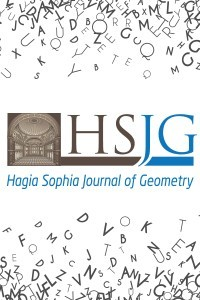e-ISSN: 2687-4261
Founded: 2019
Period: Biannually
Publisher: Salim YÜCEHagia Sophia Journal of Geometry (HSJG) publishes original research and survey papers in the fields of geometry and all interdisciplinary areas of Mathematics which use geometric methods and investigate geometrical structures. HSJG covers the following main areas: differential geometry, manifolds, Lie groups, geometric algebra, finite geometries, combinatorial geometry, kinematic geometry, Euclidean geometry, non-Euclidean geometries, matrix theory, quantum groups, Hopf algebra and and Clifford algebra.

Latest Issues

### 2021 - Volume: 3 Issue: 2

Research Article

1. On Spacelike $(1,3)$-Bertrand Curves in $E_{2}^{4}$

### Creative Commons